Theory and Modern Applications

Dynamics of a fourth-order system of rational difference equations

Abstract

In this paper, we study the equilibrium points, local asymptotic stability of an equilibrium point, instability of equilibrium points, periodicity behavior of positive solutions, and global character of an equilibrium point of a fourth-order system of rational difference equations of the form

${x}_{n+1}=\frac{\alpha {x}_{n-3}}{\beta +\gamma {y}_{n}{y}_{n-1}{y}_{n-2}{y}_{n-3}},\phantom{\rule{2em}{0ex}}{y}_{n+1}=\frac{{\alpha }_{1}{y}_{n-3}}{{\beta }_{1}+{\gamma }_{1}{x}_{n}{x}_{n-1}{x}_{n-2}{x}_{n-3}},$

$n=0,1,\dots$ , where the parameters α, β, γ, ${\alpha }_{1}$, ${\beta }_{1}$, ${\gamma }_{1}$ and initial conditions ${x}_{0}$, ${x}_{-1}$, ${x}_{-2}$, ${x}_{-3}$, ${y}_{0}$, ${y}_{-1}$, ${y}_{-2}$, ${y}_{-3}$ are positive real numbers. Some numerical examples are given to verify our theoretical results.

MSC:39A10, 40A05.

1 Introduction and preliminaries

The theory of discrete dynamical systems and difference equations developed greatly during the last twenty-five years of the twentieth century. Applications of difference equations also experienced enormous growth in many areas. Many applications of discrete dynamical systems and difference equations have appeared recently in the areas of biology, economics, physics, resource management, and others. The theory of difference equations occupies a central position in applicable analysis. There is no doubt that the theory of difference equations will continue to play an important role in mathematics as a whole. Nonlinear difference equations of order greater than one are of paramount importance in applications. Such equations also appear naturally as discrete analogues and as numerical solutions of differential and delay differential equations which model various diverse phenomena in biology, ecology, physiology, physics, engineering and economics. It is very interesting to investigate the behavior of solutions of a system of higher-order rational difference equations and to discuss the local asymptotic stability of their equilibrium points.

Cinar  investigated the periodicity of the positive solutions of the system of rational difference equations

${x}_{n+1}=\frac{1}{{y}_{n}},\phantom{\rule{2em}{0ex}}{y}_{n+1}=\frac{{y}_{n}}{{x}_{n-1}{y}_{n-1}}.$

Stević  studied the system of two nonlinear difference equations

${x}_{n+1}=\frac{{u}_{n}}{1+{v}_{n}},\phantom{\rule{2em}{0ex}}{y}_{n+1}=\frac{{w}_{n}}{1+{s}_{n}},$

where ${u}_{n}$, ${v}_{n}$, ${w}_{n}$, ${s}_{n}$ are some sequences ${x}_{n}$ or ${y}_{n}$.

Kurbanli  studied the behavior of positive solutions of the system of rational difference equations

${x}_{n+1}=\frac{{x}_{n-1}}{{y}_{n}{x}_{n-1}-1},\phantom{\rule{2em}{0ex}}{y}_{n+1}=\frac{{y}_{n-1}}{{x}_{n}{y}_{n-1}-1},\phantom{\rule{2em}{0ex}}{z}_{n+1}=\frac{1}{{y}_{n}{z}_{n}}.$

Bajo and Liz  investigated the global behavior of the difference equation

${x}_{n+1}=\frac{{x}_{n-1}}{a+b{x}_{n-1}{x}_{n}}$

for all values of real parameters a, b.

Kalabuŝić, Kulenović, and Pilav  investigated the global dynamics of the following systems of difference equations:

${x}_{n+1}=\frac{{\alpha }_{1}+{\beta }_{1}{x}_{n}}{{A}_{1}+{y}_{n}},\phantom{\rule{2em}{0ex}}{y}_{n+1}=\frac{{\gamma }_{2}{y}_{n}}{{A}_{2}+{B}_{2}{x}_{n}+{y}_{n}}.$

Kurbanli, Çinar, and Yalçinkaya  studied the behavior of positive solutions of the system of rational difference equations

${x}_{n+1}=\frac{{x}_{n-1}}{{y}_{n}{x}_{n-1}+1},\phantom{\rule{2em}{0ex}}{y}_{n+1}=\frac{{y}_{n-1}}{{x}_{n}{y}_{n-1}+1}.$

Touafek and Elsayed  studied the periodic nature and got the form of the solutions of the following systems of rational difference equations:

${x}_{n+1}=\frac{{x}_{n-3}}{±1±{x}_{n-3}{y}_{n-1}},\phantom{\rule{2em}{0ex}}{y}_{n+1}=\frac{{y}_{n-3}}{±1±{y}_{n-3}{x}_{n-1}}.$

Similarly, Touafek, and Elsayed  studied the periodicity nature of the following systems of rational difference equations:

${x}_{n+1}=\frac{{y}_{n}}{{x}_{n-1}\left(±1±{y}_{n}\right)},\phantom{\rule{2em}{0ex}}{y}_{n+1}=\frac{{x}_{n}}{{y}_{n-1}\left(±1±{x}_{n}\right)}.$

Recently, Zhang, Yang, and Liu  studied the dynamics of a system of the rational third-order difference equation

${x}_{n+1}=\frac{{x}_{n-2}}{B+{y}_{n}{y}_{n-1}{y}_{n-2}},\phantom{\rule{2em}{0ex}}{y}_{n+1}=\frac{{y}_{n-2}}{A+{x}_{n}{x}_{n-1}{x}_{n-2}},\phantom{\rule{1em}{0ex}}n=0,1,\dots .$

Our aim in this paper is to investigate the dynamics of a system of fourth-order rational difference equations

${x}_{n+1}=\frac{\alpha {x}_{n-3}}{\beta +\gamma {y}_{n}{y}_{n-1}{y}_{n-2}{y}_{n-3}},\phantom{\rule{2em}{0ex}}{y}_{n+1}=\frac{{\alpha }_{1}{y}_{n-3}}{{\beta }_{1}+{\gamma }_{1}{x}_{n}{x}_{n-1}{x}_{n-2}{x}_{n-3}},$
(1.1)

$n=0,1,\dots$ , where the parameters α, β, γ, ${\alpha }_{1}$, ${\beta }_{1}$, ${\gamma }_{1}$ and initial conditions ${x}_{0}$, ${x}_{-1}$, ${x}_{-2}$, ${x}_{-3}$, ${y}_{0}$, ${y}_{-1}$, ${y}_{-2}$, ${y}_{-3}$ are positive real numbers. This paper is a natural extension of [9, 10].

Let us consider an eight-dimensional discrete dynamical system of the form

$\begin{array}{r}{x}_{n+1}=f\left({x}_{n},{x}_{n-1},{x}_{n-2},{x}_{n-3},{y}_{n},{y}_{n-1},{y}_{n-2},{y}_{n-3}\right),\\ {y}_{n+1}=g\left({x}_{n},{x}_{n-1},{x}_{n-2},{x}_{n-3},{y}_{n},{y}_{n-1},{y}_{n-2},{y}_{n-3}\right),\end{array}$
(1.2)

$n=0,1,\dots$ , where $f:{I}^{4}×{J}^{4}\to I$ and $g:{I}^{4}×{J}^{4}\to J$ are continuously differentiable functions and I, J are some intervals of real numbers. Furthermore, a solution ${\left\{\left({x}_{n},{y}_{n}\right)\right\}}_{n=-3}^{\mathrm{\infty }}$ of the system (1.2) is uniquely determined by initial conditions $\left({x}_{i},{y}_{i}\right)\in I×J$ for $i\in \left\{-3,-2,-1,0\right\}$. Along with the system (1.2), we consider the corresponding vector map $F=\left(f,{x}_{n},{x}_{n-1},{x}_{n-2},{x}_{n-3},g,{y}_{n},{y}_{n-1},{y}_{n-2},{y}_{n-3}\right)$. An equilibrium point of (1.2) is a point $\left(\overline{x},\overline{y}\right)$ that satisfies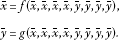The point $\left(\overline{x},\overline{y}\right)$ is also called a fixed point of the vector map F.

Definition 1.1 Let $\left(\overline{x},\overline{y}\right)$ be an equilibrium point of the system (1.2).

1. (i)

An equilibrium point $\left(\overline{x},\overline{y}\right)$ is said to be stable if for every $\epsilon >0$, there exists $\delta >0$ such that for every initial condition $\left({x}_{i},{y}_{i}\right)$, $i\in \left\{-3,-2,-1,0\right\}$ if $\parallel {\sum }_{i=-3}^{0}\left({x}_{i},{y}_{i}\right)-\left(\overline{x},\overline{y}\right)\parallel <\delta$ implies $\parallel \left({x}_{n},{y}_{n}\right)-\left(\overline{x},\overline{y}\right)\parallel <\epsilon$ for all $n>0$, where $\parallel \cdot \parallel$ is the usual Euclidean norm in ${\mathbb{R}}^{2}$.

2. (ii)

An equilibrium point $\left(\overline{x},\overline{y}\right)$ is said to be unstable if it is not stable.

3. (iii)

An equilibrium point $\left(\overline{x},\overline{y}\right)$ is said to be asymptotically stable if there exists $\eta >0$ such that $\parallel {\sum }_{i=-3}^{0}\left({x}_{i},{y}_{i}\right)-\left(\overline{x},\overline{y}\right)\parallel <\eta$ and $\left({x}_{n},{y}_{n}\right)\to \left(\overline{x},\overline{y}\right)$ as $n\to \mathrm{\infty }$.

4. (iv)

An equilibrium point $\left(\overline{x},\overline{y}\right)$ is called a global attractor if $\left({x}_{n},{y}_{n}\right)\to \left(\overline{x},\overline{y}\right)$ as $n\to \mathrm{\infty }$.

5. (v)

An equilibrium point $\left(\overline{x},\overline{y}\right)$ is called an asymptotic global attractor if it is a global attractor and stable.

Definition 1.2 Let $\left(\overline{x},\overline{y}\right)$ be an equilibrium point of the map

$F=\left(f,{x}_{n},{x}_{n-1},{x}_{n-2},{x}_{n-3},g,{y}_{n},{y}_{n-1},{y}_{n-2},{y}_{n-3}\right),$

where f and g are continuously differentiable functions at $\left(\overline{x},\overline{y}\right)$. The linearized system of (1.2) about the equilibrium point $\left(\overline{x},\overline{y}\right)$ is

${X}_{n+1}=F\left({X}_{n}\right)={F}_{J}{X}_{n},$

where

${X}_{n}=\left(\begin{array}{c}{x}_{n}\\ {x}_{n-1}\\ {x}_{n-2}\\ {x}_{n-3}\\ {y}_{n}\\ {y}_{n-1}\\ {y}_{n-2}\\ {y}_{n-3}\end{array}\right)$

and ${F}_{J}$ is a Jacobian matrix of the system (1.2) about the equilibrium point $\left(\overline{x},\overline{y}\right)$.

To construct the corresponding linearized form of the system (1.1), we consider the following transformation:

$\left({x}_{n},{x}_{n-1},{x}_{n-2},{x}_{n-3},{y}_{n},{y}_{n-1},{y}_{n-2},{y}_{n-3}\right)↦\left(f,{f}_{1},{f}_{2},{f}_{3},{f}_{4},g,{g}_{1},{g}_{2},{g}_{3},{g}_{4}\right),$
(1.3)

where $f=\frac{\alpha {x}_{n-3}}{\beta +\gamma {y}_{n}{y}_{n-1}{y}_{n-2}{y}_{n-3}}$, $g=\frac{{\alpha }_{1}{y}_{n-3}}{{\beta }_{1}+{\gamma }_{1}{x}_{n}{x}_{n-1}{x}_{n-2}{x}_{n-3}}$, ${f}_{1}={x}_{n}$, ${f}_{2}={x}_{n-1}$, ${f}_{3}={x}_{n-2}$, ${f}_{4}={x}_{n-3}$, ${g}_{1}={y}_{n}$, ${g}_{2}={y}_{n-1}$, ${g}_{3}={y}_{n-2}$ and ${g}_{4}={y}_{n-3}$. The Jacobian matrix about the fixed point $\left(\overline{x},\overline{y}\right)$ under the transformation (1.3) is given by

${F}_{J}\left(\overline{x},\overline{y}\right)=\left(\begin{array}{cccccccc}0& 0& 0& A& B& B& B& B\\ 1& 0& 0& 0& 0& 0& 0& 0\\ 0& 1& 0& 0& 0& 0& 0& 0\\ 0& 0& 1& 0& 0& 0& 0& 0\\ C& C& C& C& 0& 0& 0& D\\ 0& 0& 0& 0& 1& 0& 0& 0\\ 0& 0& 0& 0& 0& 1& 0& 0\\ 0& 0& 0& 0& 0& 0& 1& 0\end{array}\right),$

where $A=\frac{\alpha }{\beta +\gamma {\overline{y}}^{4}}$, $B=-\frac{\alpha \gamma \overline{x}{\overline{y}}^{3}}{{\left(\beta +\gamma {\overline{y}}^{4}\right)}^{2}}$, $C=-\frac{{\alpha }_{1}{\gamma }_{1}\overline{y}{\overline{x}}^{3}}{{\left({\beta }_{1}+{\gamma }_{1}{\overline{x}}^{4}\right)}^{2}}$ and $D=\frac{{\alpha }_{1}}{{\beta }_{1}+{\gamma }_{1}{\overline{x}}^{4}}$.

Theorem 1.3 For the system ${X}_{n+1}=F\left({X}_{n}\right)$, $n=0,1,\dots$ , of difference equations such that $\overline{X}$ is a fixed point of F. If all eigenvalues of the Jacobian matrix ${J}_{F}$ about $\overline{X}$ lie inside the open unit disk $|\lambda |<1$, then $\overline{X}$ is locally asymptotically stable. If one of them has a modulus greater than one, then $\overline{X}$ is unstable.

Theorem 1.4 (Routh-Hurwitz criterion)

For real numbers ${a}_{1},{a}_{2},\dots ,{a}_{n}$, let

$P\left(\lambda \right)={\lambda }^{n}+{a}_{1}{\lambda }^{n-1}+\cdots +{a}_{n-1}\lambda +{a}_{n}.$
(1.4)

Consider the polynomial equation

$P\left(\lambda \right)=0.$
(1.5)

We define the n matrices as follows: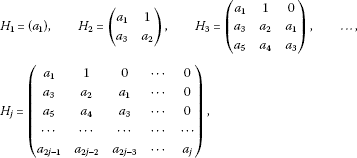where $\left(l,m\right)$ element in the matrix ${H}_{j}$, for $0<2l-m is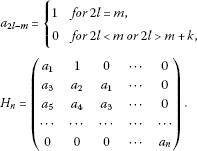The following statements are true:

1. (i)

A necessary and sufficient condition for all of the roots of (1.5) to have a negative real part is $det\left({H}_{j}\right)>0$ for $j=1,2,\dots ,n$.

2. (ii)

A necessary and sufficient condition for the existence of a root of (1.5) with a positive real part is $det\left({H}_{j}\right)<0$ for some $j\in \left\{1,2,\dots ,n\right\}$.

2 Main results

Let $\left(\overline{x},\overline{y}\right)$ be an equilibrium point of the system (1.1), then for $\alpha >\beta$ and ${\alpha }_{1}>{\beta }_{1}$, the system (1.1) has the following five equilibrium points:

${P}_{0}=\left(0,0\right),\phantom{\rule{2em}{0ex}}{P}_{1}=\left(A,B\right),\phantom{\rule{2em}{0ex}}{P}_{2}=\left(-A,B\right),\phantom{\rule{2em}{0ex}}{P}_{3}=\left(A,-B\right),\phantom{\rule{2em}{0ex}}{P}_{4}=\left(-A,-B\right),$

where $A={\left(\frac{{\alpha }_{1}-{\beta }_{1}}{{\gamma }_{1}}\right)}^{\frac{1}{4}}$ and $B={\left(\frac{\alpha -\beta }{\gamma }\right)}^{\frac{1}{4}}$.

Theorem 2.1 Let $\left({x}_{n},{y}_{n}\right)$ be a positive solution of the system (1.1), then for every $m\ge 0$, the following results hold:Proof The results are obviously true for $m=0$. Suppose that results are true for $m=k\ge 1$, i.e.,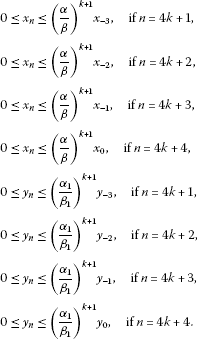Now, for $m=k+1$ using (1.1), one has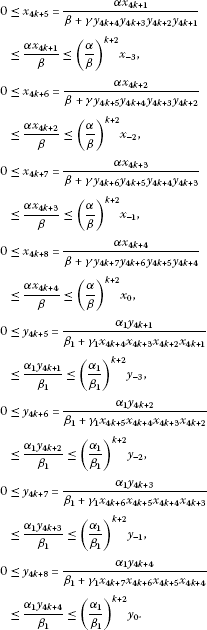□

Theorem 2.2 For the equilibrium point ${P}_{0}=\left(0,0\right)$ of Equation (1.1), the following results hold:

1. (i)

Let $\alpha <\beta$ and ${\alpha }_{1}<{\beta }_{1}$, then the equilibrium point ${P}_{0}=\left(0,0\right)$ of the system (1.1) is locally asymptotically stable.

2. (ii)

If $\alpha >\beta$ or ${\alpha }_{1}>{\beta }_{1}$, then the equilibrium point ${P}_{0}=\left(0,0\right)$ of the system (1.1) is unstable.

Proof (i) The linearized system of (1.1) about the equilibrium point $\left(0,0\right)$ is given by

${X}_{n+1}={F}_{J}\left(0,0\right){X}_{n},$

where

${X}_{n}=\left(\begin{array}{c}{x}_{n}\\ {x}_{n-1}\\ {x}_{n-2}\\ {x}_{n-3}\\ {y}_{n}\\ {y}_{n-1}\\ {y}_{n-2}\\ {y}_{n-3}\end{array}\right)$

and

${F}_{J}\left(0,0\right)=\left(\begin{array}{cccccccc}0& 0& 0& \frac{\alpha }{\beta }& 0& 0& 0& 0\\ 1& 0& 0& 0& 0& 0& 0& 0\\ 0& 1& 0& 0& 0& 0& 0& 0\\ 0& 0& 1& 0& 0& 0& 0& 0\\ 0& 0& 0& 0& 0& 0& 0& \frac{{\alpha }_{1}}{{\beta }_{1}}\\ 0& 0& 0& 0& 1& 0& 0& 0\\ 0& 0& 0& 0& 0& 1& 0& 0\\ 0& 0& 0& 0& 0& 0& 1& 0\end{array}\right).$

The characteristic polynomial of ${F}_{J}\left(0,0\right)$ is given by

$P\left(\lambda \right)={\lambda }^{8}-\left(\frac{\alpha }{\beta }+\frac{{\alpha }_{1}}{{\beta }_{1}}\right){\lambda }^{4}-\frac{\alpha {\alpha }_{1}}{\beta {\beta }_{1}}.$
(2.1)

The roots of $P\left(\lambda \right)$ are $\lambda =±\frac{\alpha }{\beta }$, $\lambda =±\frac{{\alpha }_{1}}{{\beta }_{1}}$, $\lambda =±\iota \frac{\alpha }{\beta }$, $\lambda =±\iota \frac{{\alpha }_{1}}{{\beta }_{1}}$. Since all eigenvalues of the Jacobian matrix ${F}_{J}\left(0,0\right)$ about $\left(0,0\right)$ lie in an open unit dick $|\lambda |<1$, the equilibrium point $\left(0,0\right)$ is locally asymptotically stable.

1. (ii)

It is easy to see that if $\alpha >\beta$ or ${\alpha }_{1}>{\beta }_{1}$, then there exists at least one root λ of Equation (2.1) such that $|\lambda |>1$. Hence, by Theorem 1.3 if $\alpha >\beta$ or ${\alpha }_{1}>{\beta }_{1}$, then $\left(0,0\right)$ is unstable. □

Theorem 2.3 If $\alpha >\beta$ and ${\alpha }_{1}>{\beta }_{1}$, then a positive equilibrium point ${P}_{1}=\left({\left(\frac{{\alpha }_{1}-{\beta }_{1}}{{\gamma }_{1}}\right)}^{\frac{1}{4}},{\left(\frac{\alpha -\beta }{\gamma }\right)}^{\frac{1}{4}}\right)$ of Equation (1.1) is unstable.

Proof The linearized system of (1.1) about the equilibrium point ${P}_{1}$ is given by

${X}_{n+1}={F}_{J}\left({P}_{1}\right){X}_{n},$

where

${X}_{n}=\left(\begin{array}{c}{x}_{n}\\ {x}_{n-1}\\ {x}_{n-2}\\ {x}_{n-3}\\ {y}_{n}\\ {y}_{n-1}\\ {y}_{n-2}\\ {y}_{n-3}\end{array}\right)$

and

${F}_{J}\left({P}_{1}\right)=\left(\begin{array}{cccccccc}0& 0& 0& 1& L& L& L& L\\ 1& 0& 0& 0& 0& 0& 0& 0\\ 0& 1& 0& 0& 0& 0& 0& 0\\ 0& 0& 1& 0& 0& 0& 0& 0\\ M& M& M& M& 0& 0& 0& 1\\ 0& 0& 0& 0& 1& 0& 0& 0\\ 0& 0& 0& 0& 0& 1& 0& 0\\ 0& 0& 0& 0& 0& 0& 1& 0\end{array}\right),$

where

$L=-{\left(\frac{{\gamma }_{1}}{\gamma }\right)}^{\frac{1}{4}}\frac{{\left({\alpha }_{1}-{\beta }_{1}\right)}^{\frac{1}{4}{\left(\alpha -\beta \right)}^{\frac{3}{4}}}}{\alpha }$

and

$M=-{\left(\frac{\gamma }{{\gamma }_{1}}\right)}^{\frac{1}{4}}\frac{{\left(\alpha -\beta \right)}^{\frac{1}{4}{\left({\alpha }_{1}-{\beta }_{1}\right)}^{\frac{3}{4}}}}{{\alpha }_{1}}.$

The characteristic polynomial of ${F}_{J}\left({P}_{1}\right)$ is given by

$P\left(\lambda \right)={\lambda }^{8}-LM{\lambda }^{6}-2LM{\lambda }^{5}-3LM{\lambda }^{4}-4LM{\lambda }^{3}-3LM{\lambda }^{2}-2LM\lambda -LM+1.$
(2.2)

The roots of the characteristic polynomial $P\left(\lambda \right)$ given in Equation (2.2) are given by

$-1,\phantom{\rule{2em}{0ex}}±\iota ,\phantom{\rule{2em}{0ex}}1±\sqrt{L}\sqrt{M}.$

It is sufficient to prove that any one of these roots has absolute value greater than one. For this, consider

$\begin{array}{rcl}|1-\sqrt{L}\sqrt{M}|& =& |1+\sqrt{\left(\frac{\alpha -\beta }{\alpha }\right)\left(\frac{{\alpha }_{1}-{\beta }_{1}}{{\alpha }_{1}}\right)}|\\ =& |1+\sqrt{\left(1-\frac{\beta }{\alpha }\right)\left(1-\frac{{\beta }_{1}}{{\alpha }_{1}}\right)}|>1.\end{array}$

Hence, by Theorem 1.3 if $\alpha >\beta$ and ${\alpha }_{1}>{\beta }_{1}$, then ${P}_{1}$ is unstable. □

Theorem 2.4 If $\alpha >\beta$ and ${\alpha }_{1}>{\beta }_{1}$, then the equilibrium points ${P}_{2}$, ${P}_{3}$, ${P}_{4}$ of Equation (1.1) are unstable.

Proof The proof is similar to Theorem 2.3, so it is omitted. □

The following theorem is similar to Theorem 3.4 of .

Theorem 2.5 Let $\alpha >\beta$ and ${\alpha }_{1}>{\beta }_{1}$, and let $\left({x}_{n},{y}_{n}\right)$ be a solution of the system (1.1). Then, for $k=-3,-2,-1,0$, the following statements are true:

1. (i)

If $\left({x}_{k},{y}_{k}\right)\in \left(0,{\left(\frac{{\alpha }_{1}-{\beta }_{1}}{{\gamma }_{1}}\right)}^{\frac{1}{4}}\right)×\left({\left(\frac{\alpha -\beta }{\gamma }\right)}^{\frac{1}{4}},\mathrm{\infty }\right)$, then $\left({x}_{n},{y}_{n}\right)\in \left(0,{\left(\frac{{\alpha }_{1}-{\beta }_{1}}{{\gamma }_{1}}\right)}^{\frac{1}{4}}\right)×\left({\left(\frac{\alpha -\beta }{\gamma }\right)}^{\frac{1}{4}},\mathrm{\infty }\right)$.

2. (ii)

If $\left({x}_{k},{y}_{k}\right)\in \left({\left(\frac{{\alpha }_{1}-{\beta }_{1}}{{\gamma }_{1}}\right)}^{\frac{1}{4}},\mathrm{\infty }\right)×\left(0,{\left(\frac{\alpha -\beta }{\gamma }\right)}^{\frac{1}{4}}\right)$, then $\left({x}_{n},{y}_{n}\right)\in \left({\left(\frac{{\alpha }_{1}-{\beta }_{1}}{{\gamma }_{1}}\right)}^{\frac{1}{4}},\mathrm{\infty }\right)×\left(0,{\left(\frac{\alpha -\beta }{\gamma }\right)}^{\frac{1}{4}}\right)$.

Theorem 2.6 The system (1.1) has no prime period-two solutions.

Proof Assume that $\left({p}_{1},{q}_{1}\right),\left({p}_{2},{q}_{2}\right),\left({p}_{1},{q}_{1}\right),\dots$ is a prime period-two solution of Equation (1.1) such that ${p}_{i},{q}_{i}\ne 0$ and ${p}_{i}\ne {q}_{i}$ for $i=1,2$. Then, from the system (1.1), one has

${p}_{1}=\frac{\alpha {p}_{1}}{\beta +\gamma {\left({q}_{1}{q}_{2}\right)}^{2}},\phantom{\rule{2em}{0ex}}{p}_{2}=\frac{\alpha {p}_{2}}{\beta +\gamma {\left({q}_{1}{q}_{2}\right)}^{2}},$
(2.3)

and

${q}_{1}=\frac{{\alpha }_{1}{q}_{1}}{{\beta }_{1}+{\gamma }_{1}{\left({p}_{1}{p}_{2}\right)}^{2}},\phantom{\rule{2em}{0ex}}{q}_{2}=\frac{{\alpha }_{1}{q}_{2}}{{\beta }_{1}+{\gamma }_{1}{\left({p}_{1}{p}_{2}\right)}^{2}}.$
(2.4)

From (2.3) and (2.4), one has ${p}_{i},{q}_{i}=0$ for $i=1,2$. Which is a contradiction. Hence, the system (1.1) has no prime period-two solutions. □

Theorem 2.7 Let $\alpha <\beta$ and ${\alpha }_{1}<{\beta }_{1}$, then the equilibrium point ${P}_{0}=\left(0,0\right)$ of Equation (1.1) is globally asymptotically stable.

Proof For $\alpha <\beta$ and ${\alpha }_{1}<{\beta }_{1}$, from Theorem 2.2, $\left(0,0\right)$ is locally asymptotically stable. From Theorem 2.1, it is easy to see that every positive solution $\left({x}_{n},{y}_{n}\right)$ is bounded, i.e., $0\le {x}_{n}\le \mu$ and $0\le {y}_{n}\le \nu$ for all $n=0,1,2,\dots$ , where $\mu =max\left\{{x}_{-3},{x}_{-2},{x}_{-1},{x}_{0}\right\}$ and $\nu =max\left\{{y}_{-3},{y}_{-2},{y}_{-1},{y}_{0}\right\}$. Now, it is sufficient to prove that $\left({x}_{n},{y}_{n}\right)$ is decreasing. From the system (1.1), one has

$\begin{array}{rcl}{x}_{n+1}& =& \frac{\alpha {x}_{n-3}}{\beta +\gamma {y}_{n}{y}_{n-1}{y}_{n-2}{y}_{n-3}}\\ \le & \frac{\alpha {x}_{n-3}}{\beta }<{x}_{n-3}.\end{array}$

This implies that ${x}_{4n+1}<{x}_{4n-3}$ and ${x}_{4n+5}<{x}_{4n+1}$. Hence, the subsequences $\left\{{x}_{4n+1}\right\}$, $\left\{{x}_{4n+2}\right\}$, $\left\{{x}_{4n+3}\right\}$, $\left\{{x}_{4n+4}\right\}$ are decreasing, i.e., the sequence $\left\{{x}_{n}\right\}$ is decreasing. Similarly, one has

$\begin{array}{rcl}{y}_{n+1}& =& \frac{{\alpha }_{1}{x}_{n-3}}{{\beta }_{1}+{\gamma }_{1}{y}_{n}{y}_{n-1}{y}_{n-2}{y}_{n-3}}\\ \le & \frac{{\alpha }_{1}{y}_{n-3}}{{\beta }_{1}}<{y}_{n-3}.\end{array}$

This implies that ${y}_{4n+1}<{y}_{4n-3}$ and ${y}_{4n+5}<{y}_{4n+1}$. Hence, the subsequences $\left\{{y}_{4n+1}\right\}$, $\left\{{y}_{4n+2}\right\}$, $\left\{{y}_{4n+3}\right\}$, $\left\{{y}_{4n+4}\right\}$ are decreasing, i.e., the sequence $\left\{{y}_{n}\right\}$ is decreasing. Hence, ${lim}_{n\to \mathrm{\infty }}{x}_{n}={lim}_{n\to \mathrm{\infty }}{y}_{n}=0$. □

Theorem 2.8 Let $\alpha >\beta$ and ${\alpha }_{1}>{\beta }_{1}$. Then, for a solution $\left({x}_{n},{y}_{n}\right)$ of the system (1.1), the following statements are true:

1. (i)

If ${x}_{n}\to 0$, then ${y}_{n}\to \mathrm{\infty }$.

2. (ii)

If ${y}_{n}\to 0$, then ${x}_{n}\to \mathrm{\infty }$.

3 Examples

In order to verify our theoretical results and to support our theoretical discussions, we consider several interesting numerical examples in this section. These examples represent different types of qualitative behavior of solutions to the system of nonlinear difference equations (1.1). All plots in this section are drawn with mathematica.

Example Consider the system (1.1) with initial conditions ${x}_{-3}=1.1$, ${x}_{-2}=2.6$, ${x}_{-1}=1.6$, ${x}_{0}=1.7$, ${y}_{-3}=1.5$, ${y}_{-2}=1.3$, ${y}_{-1}=2.5$, ${y}_{0}=0.5$. Moreover, choosing the parameters $\alpha =0.01$, $\beta =0.011$, $\gamma =50$, ${\alpha }_{1}=0.03$, ${\beta }_{1}=0.031$, ${\gamma }_{1}=70$, the system (1.1) can be written as follows:

${x}_{n+1}=\frac{0.01{x}_{n-3}}{0.011+50{y}_{n}{y}_{n-1}{y}_{n-2}{y}_{n-3}},\phantom{\rule{2em}{0ex}}{y}_{n+1}=\frac{0.03{y}_{n-3}}{0.031+70{x}_{n}{x}_{n-1}{x}_{n-2}{x}_{n-3}},$
(3.1)

$n=0,1,\dots$ , and with initial conditions ${x}_{-3}=1.1$, ${x}_{-2}=2.6$, ${x}_{-1}=1.6$, ${x}_{0}=1.7$, ${y}_{-3}=1.5$, ${y}_{-2}=1.3$, ${y}_{-1}=2.5$, ${y}_{0}=0.5$. The plot of the system (3.1) is shown in Figure 1 and its global attractor is shown in Figure 2.

Example Consider the system (1.1) with initial conditions ${x}_{-3}=2.1$, ${x}_{-2}=2.6$, ${x}_{-1}=0.6$, ${x}_{0}=1.4$, ${y}_{-3}=1.5$, ${y}_{-2}=1.6$, ${y}_{-1}=2.7$, ${y}_{0}=0.45$. Moreover, choosing the parameters $\alpha =12$, $\beta =12.5$, $\gamma =90$, ${\alpha }_{1}=15$, ${\beta }_{1}=15.5$, ${\gamma }_{1}=75$, the system (1.1) can be written as follows:

${x}_{n+1}=\frac{12{x}_{n-3}}{12.5+90{y}_{n}{y}_{n-1}{y}_{n-2}{y}_{n-3}},\phantom{\rule{2em}{0ex}}{y}_{n+1}=\frac{15{y}_{n-3}}{15.5+75{x}_{n}{x}_{n-1}{x}_{n-2}{x}_{n-3}},$
(3.2)

$n=0,1,\dots$ , and with initial conditions ${x}_{-3}=2.1$, ${x}_{-2}=2.6$, ${x}_{-1}=0.6$, ${x}_{0}=1.4$, ${y}_{-3}=1.5$, ${y}_{-2}=1.6$, ${y}_{-1}=2.7$, ${y}_{0}=0.45$. The plot of the system (3.2) is shown in Figure 3 and its global attractor is shown in Figure 4.

Example Consider the system (1.1) with initial conditions ${x}_{-3}=9.2$, ${x}_{-2}=1.8$, ${x}_{-1}=0.76$, ${x}_{0}=1.1$, ${y}_{-3}=1.1$, ${y}_{-2}=1.2$, ${y}_{-1}=8.1$, ${y}_{0}=0.52$. Moreover, choosing the parameters $\alpha =200$, $\beta =225$, $\gamma =1000$, ${\alpha }_{1}=150$, ${\beta }_{1}=160$, ${\gamma }_{1}=700$, the system (1.1) can be written as follows:

${x}_{n+1}=\frac{200{x}_{n-3}}{225+1000{y}_{n}{y}_{n-1}{y}_{n-2}{y}_{n-3}},\phantom{\rule{2em}{0ex}}{y}_{n+1}=\frac{150{y}_{n-3}}{160+700{x}_{n}{x}_{n-1}{x}_{n-2}{x}_{n-3}},$
(3.3)

$n=0,1,\dots$ , and with initial conditions ${x}_{-3}=9.2$, ${x}_{-2}=1.8$, ${x}_{-1}=0.76$, ${x}_{0}=1.1$, ${y}_{-3}=1.1$, ${y}_{-2}=1.2$, ${y}_{-1}=8.1$, ${y}_{0}=0.52$. The plot of the system (3.3) is shown in Figure 5 and its global attractor is shown in Figure 6.

Example Consider the system (1.1) with initial conditions ${x}_{-3}=1.3$, ${x}_{-2}=1.8$, ${x}_{-1}=2.6$, ${x}_{0}=2.1$, ${y}_{-3}=0.01$, ${y}_{-2}=1.2$, ${y}_{-1}=2.8$, ${y}_{0}=1.5$. Moreover, choosing the parameters $\alpha =12$, $\beta =10.5$, $\gamma =15$, ${\alpha }_{1}=14$, ${\beta }_{1}=13$, ${\gamma }_{1}=0.2$, the system (1.1) can be written as follows:

${x}_{n+1}=\frac{12{x}_{n-3}}{10.5+15{y}_{n}{y}_{n-1}{y}_{n-2}{y}_{n-3}},\phantom{\rule{2em}{0ex}}{y}_{n+1}=\frac{14{y}_{n-3}}{13+0.2{x}_{n}{x}_{n-1}{x}_{n-2}{x}_{n-3}},$
(3.4)

$n=0,1,\dots$ , with initial conditions ${x}_{-3}=1.3$, ${x}_{-2}=1.8$, ${x}_{-1}=2.6$, ${x}_{0}=2.1$, ${y}_{-3}=0.01$, ${y}_{-2}=1.2$, ${y}_{-1}=2.8$, ${y}_{0}=1.5$. The plot of the system (3.4) is shown in Figure 7.

4 Conclusion

This work is a natural extension of [9, 10]. In the paper, we investigated some dynamics of an eight-dimensional discrete system. The system has five equilibrium points all of which except $\left(0,0\right)$ are unstable. The linearization method is used to show that the equilibrium point $\left(0,0\right)$ is locally asymptotically stable. We prove that the system has no prime period-two solutions. The main objective of dynamical systems theory is to predict the global behavior of a system based on the knowledge of its present state. An approach to this problem consists of determining the possible global behaviors of the system and determining which initial conditions lead to these long-term behaviors. In case of higher-order dynamical systems, it is crucial to discuss global behavior of the system. Some powerful tools such as semiconjugacy and weak contraction cannot be used to analyze global behavior of the system (1.1). In the paper, we prove the global asymptotic stability of the equilibrium point $\left(0,0\right)$ by using simple techniques. Some numerical examples are provided to support our theoretical results. These examples are experimental verifications of theoretical discussions.

References

1. 1.

Cinar C:On the positive solutions of the difference equation system ${x}_{n+1}=\frac{1}{{y}_{n}}$; ${y}_{n+1}=\frac{{y}_{n}}{{x}_{n-1}{y}_{n-1}}$. Appl. Math. Comput. 2004, 158: 303–305. 10.1016/j.amc.2003.08.073

2. 2.

Stević S: On some solvable systems of difference equations. Appl. Math. Comput. 2012, 218: 5010–5018. 10.1016/j.amc.2011.10.068

3. 3.

Kurbanli AS:On the behavior of positive solutions of the system of rational difference equations ${x}_{n+1}=\frac{{x}_{n-1}}{{y}_{n}{x}_{n-1}-1}$, ${y}_{n+1}=\frac{{y}_{n-1}}{{x}_{n}{y}_{n-1}-1}$, ${z}_{n+1}=\frac{1}{{y}_{n}{z}_{n}}$. Adv. Differ. Equ. 2011., 2011: Article ID 40

4. 4.

Bajo I, Liz E: Global behaviour of a second-order nonlinear difference equation. J. Differ. Equ. Appl. 2011, 17(10):1471–1486. 10.1080/10236191003639475

5. 5.

Kalabuŝić S, Kulenović MRS, Pilav E: Dynamics of a two-dimensional system of rational difference equations of Leslie–Gower type. Adv. Differ. Equ. 2011. doi:10.1186/1687–1847–2011–29

6. 6.

Kurbanli AS, Çinar C, Yalçinkaya I:On the behavior of positive solutions of the system of rational difference equations ${x}_{n+1}=\frac{{x}_{n-1}}{{y}_{n}{x}_{n-1}+1}$, ${y}_{n+1}=\frac{{y}_{n-1}}{{x}_{n}{y}_{n-1}+1}$. Math. Comput. Model. 2011, 53: 1261–1267. 10.1016/j.mcm.2010.12.009

7. 7.

Touafek N, Elsayed EM: On the solutions of systems of rational difference equations. Math. Comput. Model. 2012, 55: 1987–1997. 10.1016/j.mcm.2011.11.058

8. 8.

Touafek N, Elsayed EM: On the periodicity of some systems of nonlinear difference equations. Bull. Math. Soc. Sci. Math. Roumanie 2012, 2: 217–224.

9. 9.

Zhang Q, Yang L, Liu J: Dynamics of a system of rational third order difference equation. Adv. Differ. Equ. 2012. doi:10.1186/1687–1847–2012–136

10. 10.

Shojaei M, Saadati R, Adibi H: Stability and periodic character of a rational third order difference equation. Chaos Solitons Fractals 2009, 39: 1203–1209. 10.1016/j.chaos.2007.06.029

Acknowledgements

Authors would like to thank the referees for their comments and suggestions on the manuscript. This work was supported by the Higher Education Commission of Pakistan.

Author information

Authors

Corresponding author

Correspondence to Q Din.

Competing interests

The authors declare that they have no competing interests.

Authors’ contributions

QD and MNQ carried out the theoretical proof and drafted the manuscript. AQK participated in the design and coordination. All authors read and approved the final manuscript.

Authors’ original submitted files for images

Below are the links to the authors’ original submitted files for images.

Rights and permissions

Reprints and Permissions

Din, Q., Qureshi, M. & Khan, A.Q. Dynamics of a fourth-order system of rational difference equations. Adv Differ Equ 2012, 215 (2012). https://doi.org/10.1186/1687-1847-2012-215